# 流体模拟Fluid Simulation：Level Set & Marching Cube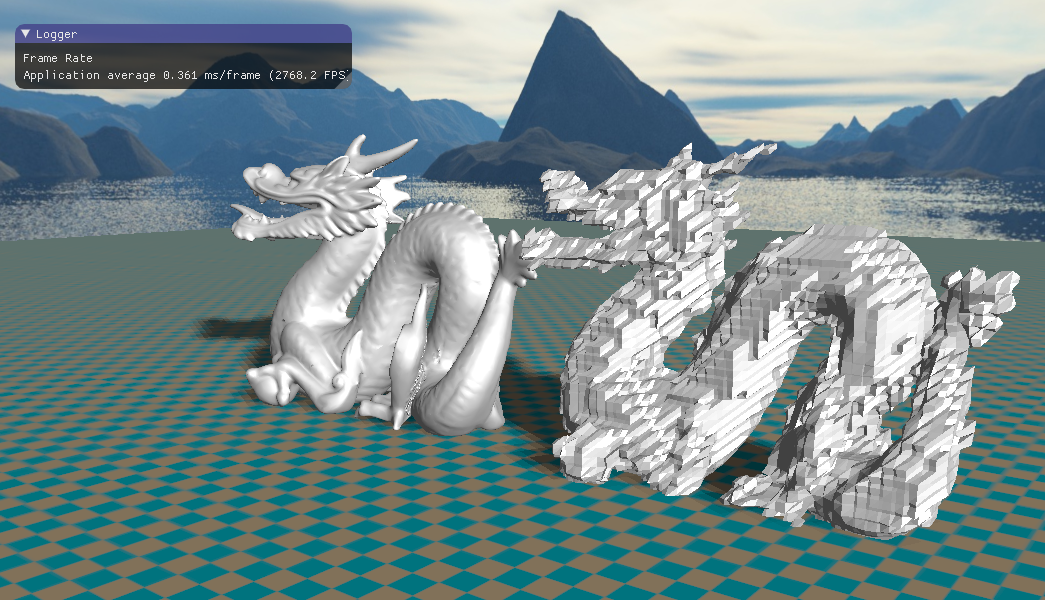• 符号距离场
• 三角形网格的SDF计算
• Marching Cube重建算法
• 参考资料

在流体模拟中，我们需要处理流体与固体边界的交互作用。这对于流体模拟来说至关重要，因为流体通常是作用到固体以及其他边界上才会体现流体的特性，让人看起来是流体。这就涉及到两个问题：

1、给定一个点（例如用半拉格朗日后向追踪得到的点），如何判断它是否在固体边界内？

2、如何计算给定一点到几何体表面上的最近的点？

上面的问题在流体模拟中非常常见，在基于欧拉网格的流体模拟中，我们常用的是水平集的方法。我们将几何体表示成一个隐式的表面，给定关于这个几何体的连续标量函数$\phi(x,y,z)$，我们定义该几何体构成的空间如下：

1、当$\phi(x,y,z)>0$时，点$x$在几何体外部，此时满足该条件的$x$的点集构成几何体的外部空间；

2、当$\phi(x,y,z) <0$时，点$x$在几何体内部，此时满足该条件的$x$的点集构成几何体的内部空间；

3、当$\phi(x,y,z)=0$时，点$x$在几何体的表面上，此时的$x$的点集构成了几何体的表面区域。

可以看到，我们采用了一个连续的标量函数$\phi(x,y,z)$来隐式地表示一个几何体，几何体的表面就是函数$\phi(x,y,z)$取值为$0$的所有点集，在三维的情况下这个点集构成了关于$\phi(x,y,z)$的一个等高面（或者说等值面），在这个等高面上$\phi(x,y,z)$取值均为$0$，这就是“水平集”一词的由来，几何体的表面就是$\phi(x,y,z)$的$0$水平集。事实上，我们要寻找的这个连续标量函数$\phi(x,y,z)$就是符号距离场（Signed Distance Field，简称SDF）。

## 一、符号距离场

任意给定一个要隐式表达的点的闭集$S$，关于这个点集$S$的距离场函数为：

该距离场函数给出了$\vec x$到点集$S$的最近距离。若点集$S$将三维空间划分成了良定义的外部和内部（例如一个封闭的三角形网格模拟），那么其符号距离场函数就定义为：

符号距离场具有需要非常有用的性质。首先是符号距离场的梯度，根据矢量微积分，梯度指向函数值变换最快的方向，在这里显然沿着法线方向距离场函数值的变换最快，因而表面上的法线向量就等于表面上的符号距离场函数的梯度$\nabla \phi(\vec x)$。在几何体的外部，$-\nabla \phi(\vec x)$指向$\vec x$到几何体表面上的最近点$\vec p$；在几何体内部，$\nabla \phi(\vec x)$指向$\vec x$到几何体表面上的最近点$\vec p$。

以外部的点$\vec x$为例，记$\vec p$为点$\vec x$到几何体表面上的最近的点，$\vec c$为点$\vec x$到最近点$\vec p$的单位方向向量：

如果我们将点$\vec x$沿着$\vec c$方向移动非常小的距离$\epsilon$，即$\vec x’=\vec x+ \epsilon \vec c$，那么点$\vec x’$到几何体表面上的最近点依然是$\vec p$。即若沿着$\vec c$方向移动$\vec x$点，其到物体表面上的最近点$\vec p$保持不变。挪动得到的新的点$\vec x’$的符号距离场函数为$\phi(\vec x’)=\phi(x)-\epsilon$，从而我们可以得出符号距离场关于$\vec c$方向向量上的方向导数为：

根据上面的推导，我们知道$\phi(\vec x)$沿着梯度方向的变化速率为$1$，从而可知梯度向量长度为$1$，这意味着SDF的梯度实际上是一个单位向量，因而表面上的单位法线就是等于SDF函数的梯度向量：

除此之外，上面的公式$(5)$是一个非线性偏微分方程——程函方程（Eikonal equation）。根据SDF函数及其梯度，我们可以求得外部的点$\vec x$到几何体表面上的距离最近的点$\vec p$：

一般情况下，符号距离场函数都是光滑的，即梯度$\nabla \phi$和更高阶的导数都存在，但是也存在一些例外的点。一些中轴上的点到几何体表面的最近距离的点可能不唯一，例如球体的圆心到球体表面最近的距离就是半径$r$，但是其最近距离上的点有无穷多个，凹几何体同样存在这种情况。在这些特定的区域，符号距离场函数依然连续，只是存在一些扭曲，但函数不可微，因而梯度以及更高阶的导数不存在。在这些不可微的区域，我们的数值近似方法依旧会给出一个梯度向量，但这个梯度向量远远小于单位向量，甚至接近于$0$。

## 二、三角形网格的SDF计算

了解了符号距离场之后，我们现在就把目标转到几何体的符号距离场计算上面。对于一些简单的几何体我们可以轻松地写出其SDF的解析表达式，例如一个球心为$\vec c$、半径为$r$的球体其SDF函数为$\phi_{sphere}(\vec x)=||\vec x-\vec c||-r$，一个以$\vec n$为法线、过点$\vec p$的无限平面其SDF函数为$\phi_{plane}(\vec x)=(\vec x-\vec p)\cdot \vec n$，还有一些简单的锥体、柱体、立方体等等都可以直接写出其SDF表达式。但是通常情况下我们接触的几何体更多的是采用三角形的网格模型来表示，三角形构成的网格模型并没有一个显式的几何函数，因而不可能像简单的几何体那样直接写出SDF的解析函数。

与直接获取几何体的解析SDF函数不一样，对于三角网格模型，我们将其所开的空间划分成一个欧拉网格，在每一个网格点上采样并存储网格模型的SDF值。然后在后面获取给定点$\vec x$的SDF函数值时，我们根据$\vec x$获取其周围网格点的SDF值，接着做一个插值操作，从而快速地获取$\vec x$对应的SDF函数值。这就是所谓的水平集方法：对符号距离场函数进行离散采样，并存储到均匀划分的网格中。显然这是求解了SDF函数的数值表达式，相对于解析表达式，它没有那么精确，但是对于流体模拟来说这些误差还是能够接受的。

我们首先要计算每个网格点上的符号距离场的值，目前主要有两种算法：第一种从几何角度展开，寻找给定的点到三角网格模型上的最近点并计算最近的距离场；第二种围绕偏微分方程展开，求解SDF的程函方程$||\nabla\phi||=1$。两种算法都有各自的用途，第一种方法更好理解，结果也更为精确，而第二中方法则适用于非显式表示的几何体。这里我们首先考虑第一种基于几何的方法。

我们首先来看一个简化了的问题：给定一个有限的点集，计算任一点$\vec x$到这个点集的距离场。由于点集并没有内部和外部的划分概念，因此我们的距离场也无需带上符号。计算的伪代码如下图1所示。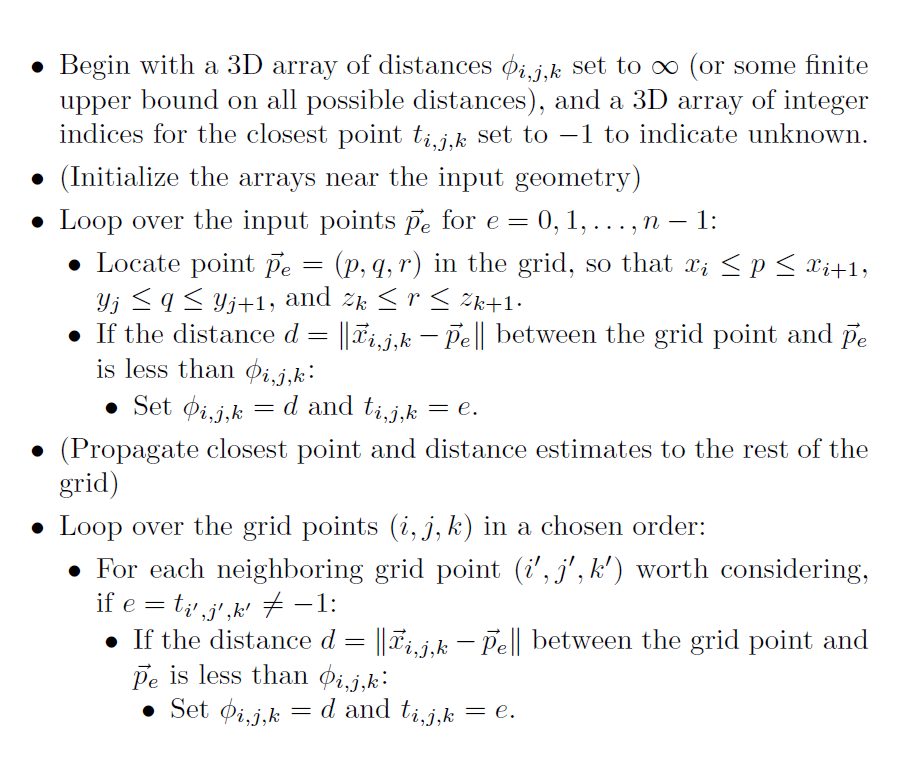算法主要分为两步，首先初始化一个三维的$\phi_{i,k,k}$数组，我们计算得到的SDF将存储到这个数组中。第一步，输入的点计算其对应的欧拉网格点，并更新该欧拉网格的SDF函数。第二步就是将第一步计算的SDF值扩散到其他未填充的欧拉网格点。上面的伪代码中第二步循环的顺序非常重要，因为这影响到其他欧拉网格点的SDF值的正确性。关于第二步的循环顺序，有两种比较推荐的方法，分别是快速移动算法和快速扫描算法，算法的复杂度分别为$O(nlog n)$和$O(n)$。我们目前仅看后一种，即快速扫描算法。

经过图1中的第一步之后，点集周围的欧拉网格点已经获取了距离场信息。第二步就是剩下的欧拉网格点需要从这些已知的欧拉网格点获取距离场信息。快速扫描算法基于这样的一个事实：最终每个未知的欧拉网格点的距离场信息必然是从其周围的某一个方向传播过来。为了确保每个欧拉网格点获取正确的距离场信息，我们遍历所有的传播方向。在三维空间中，传播方向有八个，即$i$递增、递减，$j$递增、递减，以及$k$递增、递减。因此算法将循环遍历八次，每次循环按照指定的方向进行遍历。为了提升算法的准确率，我们还可以多重复几次执行快速扫描算法。

上面我们讨论点集的水平集计算方法，接下来我们就继续深入到关于三角网格的水平集计算方法，这里的三角形网格是一个封闭的三角形网格。三角网格的水平集计算方法也是在上面点集的水平集计算方法的基础上展开，与之不同的是，这里我们追踪的不是点而是三角形。除此之外，我们还需要一些额外的步骤用于判断每个欧拉网格点是属于外部还是内部，这将影响到SDF函数值的符号。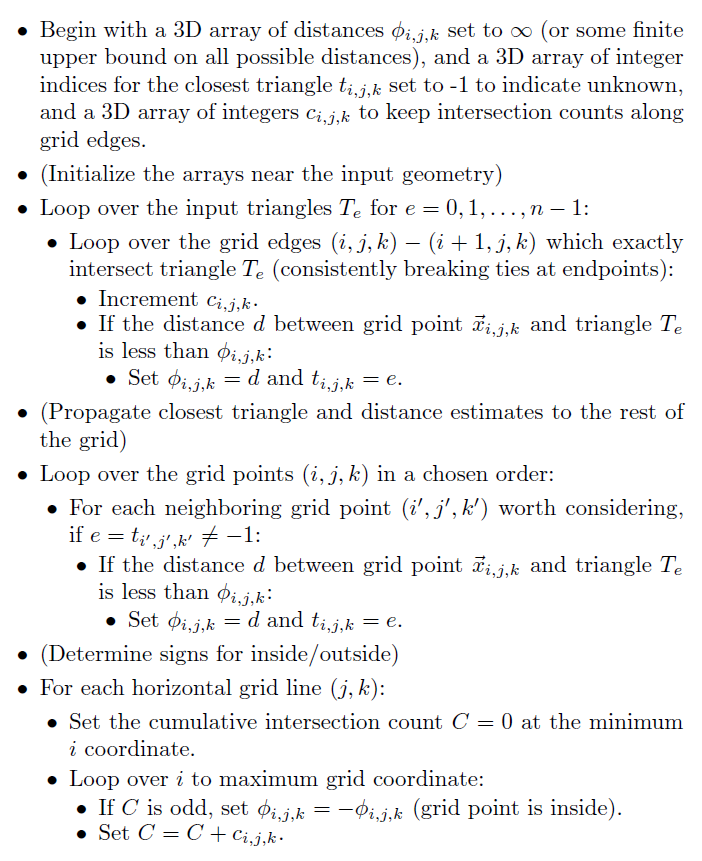三角网格的水平集计算方法比较复杂，图2的伪代码其实有些地方没有详细地说明，而这些地方恰恰非常关键。除了申请了一个三维数组用于存储$\phi_{i,j,k}$和一个三维数组存储每个最近点的索引$t_{i,j,k}$，我们还申请了一个整数数组$c_{i,j,k}$记录相交次数。为了判断一个欧拉网格点是在三角网格内部还是外部，我们采用了投射射线法：如果一个点按照某个方向发射射线，该射线与三角网格模型的交点个数为奇数个，则该点处于网格模型内部；若该射线与三角网格模型的交点个数为偶数个，则该点处于网格模型的外部。

我们向$x$轴的负方向投射射线，暴力的方法就是每一个欧拉网格点都投射一条射线，但这其实是没必要的。我们创建了一个相交次数的记录数组$c_{i,j,k}$，每一个欧拉网格点$i,j,k$检测其到$i+1,j,k$点的边是否与三角形相交，然后在算法的最后，我们将$i$从$0$开始递增，理解$c_{i,j,k}$数组，并判断当前的累加值是奇数还是偶数，从而确定距离场的符号值。检测每一条$i,j,k$到$i+1,j,k$的边与三角形的相交情况时，因为投射的射线是平行于$x$轴的，我们可以将三角形投影到$yz$平面上，然后检测$j,k$是否在投影到$yz$平面上的三角形内。若在投影的三角形内，则必然相交，反之不相交。因此，我们需要一个方法快速判断给定的点是否在二维的三角形内。

这里我们采用SoS（Simulation Of Simplicity$^{}$）的方法。给定两个二维向量$\vec a$和$\vec b$，在不考虑三维的情况下，二维的向量乘积结果为标量，其几何意义为由$\vec a$和$\vec b$构成的平行四边形的有向面积，$|\vec a\times \vec b|=|\vec a||\vec b|sin\theta$。当$\vec a\times \vec b < 0$时，两者夹角大于180度；当$\vec a\times \vec b =0$时，两者共线（可能同向，可能反向）；当$\vec a\times \vec b>0$时，两者夹角小于180度。观察下面的图3，三角形由$x_1$、$x_2$和$x_3$构成，设要判断的点为$p$，我们可得向量$\vec l_1 = {x_1-p}$、$\vec l_2 = x_2-p$以及$\vec l_3 = x_3-p$。注意到这样的一个事实，若点$p$在三角形内部，则向量$\vec l_1$、$\vec l_2$和$\vec l_3$两两之间的夹角必定小于180度。若点$p$在三角形外部，则必定（以逆时针方向为例）存在两个向量之间的夹角大于0，此时的叉乘结果小于0，正如下图中的$p_2$，逆时针方向上，$x_1-p_2$和$x_2-p_2$夹角大于0了。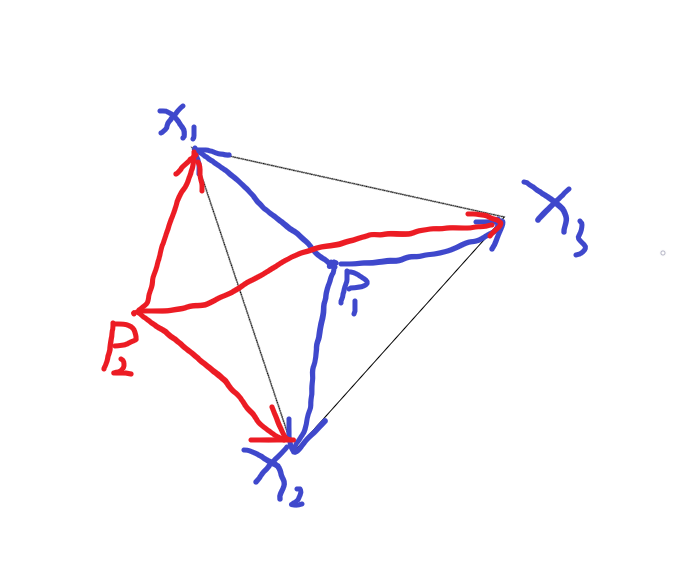上面的讨论我们已经知道如何判断给定的点是否在三角形内部了，接下来我们计算给定的点在三角形内部的重心坐标，后面我们在求交运算时需要根据重心坐标进行插值获取第三维的信息。还是一样，注意到叉乘的几何意义，我们通过叉乘得到了三个四边形的有向面积，然后除以2就是点$p$与三个顶点$x_1$、$x_2$和$x_3$构成的三角形的有向面积$S_1$、$S_2$、$S_3$，重心坐标就可以根据这些有向面积再除三角形总的有向面积和：

最后编程代码如下所示，我们首先判断三个有向面积符号是否相同，若有一个不同则直接返回不在三角形内部的情况。最后根据有向面积计算相应的重心坐标。这里的二维判断仅用于相交计算。

除了前面的二维相交计算，在三维空间中，我们还要求欧拉网格点到三角形的垂直距离，从而得到每个点的距离场。这里采用重心法求点到三角形距离。求的三角形重心坐标$\alpha$、$\beta$、$\gamma$，设三角形的三个顶点为$\vec x_1$、$\vec x_2$、$\vec x_3$，求重心坐标就是求下面的$2\times2$的矩阵方程组：

上面公式中的$\vec x_{21}$是$\vec x_2-\vec x_1$的简写，其他的类似。当且仅当求得的重心坐标$\alpha$、$\beta$和$\gamma$均大于0时，交点才在三角形的内部，此时的最近点就为$\alpha \vec x_1+\beta \vec x_2+\gamma \vec x_3$。否则SDF最近的点应该在三角形的边上，这里有一个小小的优化，就是若有一个重心坐标值大于0，则该重心坐标对应的点的对边不需要考虑进来。例如$\alpha>0$，则无需考虑$\vec x_2-\vec x_1$这条边，但通常我们需要考虑剩下的两条边，然后取两者的距离最小值。

求一点到给定线段的距离其实跟三角形的重心法类似，我们将给定的点投影到边上，然后取比值$\theta$（即线段的重心坐标）。设求点$\vec x_0$到线段$\vec x_1-\vec x_2$的最近的距离，则最近的点为$(1-\theta)\vec x_1+\vec x_2$。

解决了以上问题，接下来我们就能完成Level Set算法中的第一步了：遍历模型的所有三角形，对于每一个三角形，我们计算其包围盒，在包围盒内的所有欧拉网格点，计算其到该三角形的垂直距离并与当前自己记录的最小距离进行比较、替换，与此同时记录最近的三角形的索引；然后将三角形投影（这里的投影很简单，不需要任何的计算，直接舍弃第三维$x$的值即可）到$yz$平面，计算其二维的包围盒，在这个二维包围盒内的所有点，判断其是否在三角形内，若在三角形内，则对应的相交记录数加1。

紧接着第二步就是扩散步骤，在第一步中有些欧拉网格点已经获取了距离场信息，现在要将其传递到其它未知的欧拉网格中，从而得到完整的水平集结构。这里我们采用fast sweep算法，在三维空间中，传播方向有八个，即$i$递增、递减，$j$递增、递减，以及$k$递增、递减。因此算法将循环遍历八次，每次循环按照指定的方向进行遍历。同时，为了提高算法的准确率，我们设置迭代两次，每次传递八个方向。传播时，每个欧拉网格点根据给定的方向获取其邻居的距离场信息，若根据邻居的距离场信息得到的距离场数值更小，则进行替换。

最后的第三步就是修正距离场的符号，前面我们计算得到的距离场都是没有符号的。有了前面计算得到的相交记录数组，判断符号就容易了。注意前面我们计算得到的相交记录的值仅仅是一条边的相交记录，最后我们从$x$轴负方向朝着$x$轴正方面推进，叠加相交记录次数，得到以当前欧拉网格点为起点、朝向$x$轴负方向的射线与网格的相交情况，若为奇数，则需要将距离场的值置为负数。

## 三、Marching Cube重建算法

在前面我们实现了Level Set的构造方法，通过离散空间将三角网格的SDF值保存下来。在欧拉方法的流体模拟中，除了将三角网格模型离散化为水平集之外，还涉及到根据给定的水平集以及等高值，从中重建出网格模型，最经典的就是流体表面的网格重建过程。根据给定的欧拉网格结构重建网格模型最经典、常用的算法就是Marching Cube算法。Marching Cube算法的基本思想就是在欧拉网格的每一个立方体内，寻找在等值面上的网格点。一个立方体有八个顶点，每个顶点对应的函数值各有不同。以0等值面为例，我们要寻找函数值为0的网格点，从而生成三角网格模型。假设有一条边及其两个端点$(i,j,k)$和$(i+1,j,k)$，倘若其一端$(i,j,k)$的函数值大于0，另一端$(i+1,j,k)$的函数值小于0，那么必然在这条线段上存在一个0等值点$p$，可以通过线性插值得到：

立方体有八个顶点，给定一个值，相对于这个值，每个顶点的函数值分为大于给定值和小于给定值两种情况。因此在一个立方体上，传统的Marching Cube算法需要考虑$2^8=256$中情况，这通常通过预先生成一个查找表来进行。即便如此，Robert Brdison指出Lorensen等人的Marching Cube算法因为拓扑结构的歧义性偶尔会产生一些孔洞，从而得到非封闭的网格模型。这里我们采用Marching Cube中的一个鲁棒性更强的算法——Marching Tetrahedra算法，与Marching Cube的直接对整个立方体进行操作不同，Marching Tetrahedra算法将立方体进一步划分成多个四面体（Tetrahedra），然后对四面体进行操作，即计算四面体四个顶点的函数值，并判断是否存在等值点，存在的话就根据等值点构建三角形。

将立方体划分成多个四面体的方法如下图4所示，立方体有三对对立面，即上和下、左和右、前和后。对于每一个对立面，取同一轨迹的对角线，以这两条对角线所在的平面将立方体，如此切割三次就能得到构成立方体的六个四面体（下图4中六个不同颜色的四面体）。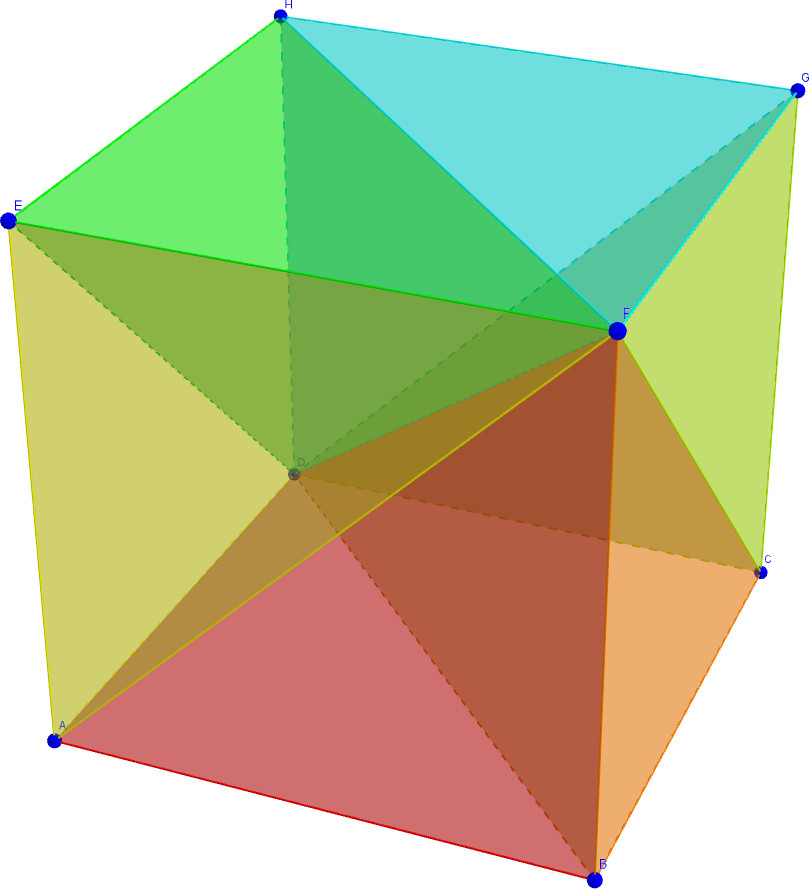与传统的Marching Cube算法相比，Marching Tetrahedra算法的优势更多。在Marching Cube算法中，操作单位为一个立方体，一个等值面截过立方体构成的截面可以是很复杂的曲面；而在Marching Tetrahedra算法中，操作单位变成了一个四面体，一个等值面截过四面体构成的截面通常只能是单个三角形、或者一个四边形（这个四边形可以分成两个三角形）。另外，因为四面体只有4个顶点，因而单个四面体只需考虑$2^4=16$中情况，相对于原来的$256$种情况大大降低了代码的复杂度。Marching Tetrahedra唯一的缺点就是生成的三角形偏多。

Marching Tetrahedra算法不太复杂，算法总体上就是一个循环，遍历所有的cube，然后将cube划分成六个四面体，根据四面体四个顶点的函数值判断是否存在三角形。上面已经提到过了，在四面体中最多就存在两个三角形。Marching Tetrahedra算法总体上分为三种情况：不存在三角形、存在一个三角形、存在两个三角形。

首先来看第一种情况，即不存在三角形。这种情况很好理解，当四面体所有顶点的函数值均为正数或者负数时（这里的正数或负数是相对于给定的函数值点来说，大于给定值则为正，小于则为负），不存在一个0等值点（或者其他等值点），从而不存在三角形。

然后是第二种情况，即存在一个三角形。注意到一个这样的事实：当四面体的一个顶点函数值为正数，其余三个顶点为负数时，在正值顶点与负值顶点相连的边上可以得到一个等值点，正值顶点分别与三个负值顶点相连的边上可以得到三个等值点，从而构成一个三角形。反过来也一样，当只有一个顶点为负数，其余顶点为正数时同理。如下图5所示，四面体的四个顶点分别为$v_1$、$v_2$、$v_3$和$v_4$，其中$v_1$的函数值为负值，$v_2$、$v_3$和$v_4$的函数值为正，从而通过线性插值得到图中的三个等值点$p_1$、$p_2$和$p_3$，将这三个顶点相连即可得到一个三角形。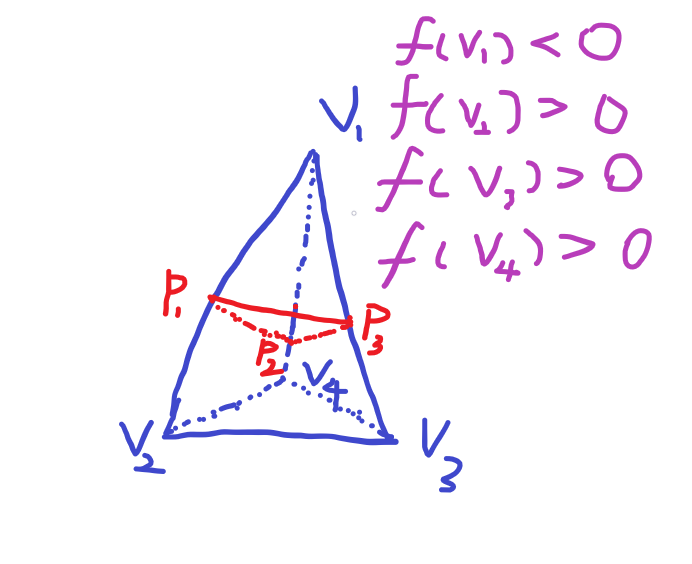一个三角形的情形共有8种，一正三负4种，一负三正4种，在代码种逐一枚举即可。这里需要注意，当三角形退化成一个点时我们不将将其纳入我们的网格顶点中。同时为了使得生成的三角形环绕顺序遵循向外的逆时针方向，在实现时需特别注意顶点的顺序。

最后就是第三种情况，即存在两个三角形。当四个顶点中有两个顶点函数值为正，两个顶点函数值为负时，从正值顶点分别于负值顶点相连的边上可以获取一个等值点，一共可以得到四个等值点，构成一个四边形，或者说两个三角形。如下图6所示，负值顶点为$v_1$、$v_2$，正值顶点为$v_3$、$v_4$，$v_1$分别与$v_3$、$v_4$相连的边上可以得到等值点$p_1$、$p_2$，$v_2$分别与$v_3$、$v_4$相连的边上可以得到等值点$p_3$、$p_4$。最后根据这四个点分别作两个三角形即可。两个三角形的情况共有$C_4^2=6$种，逐一枚举即可。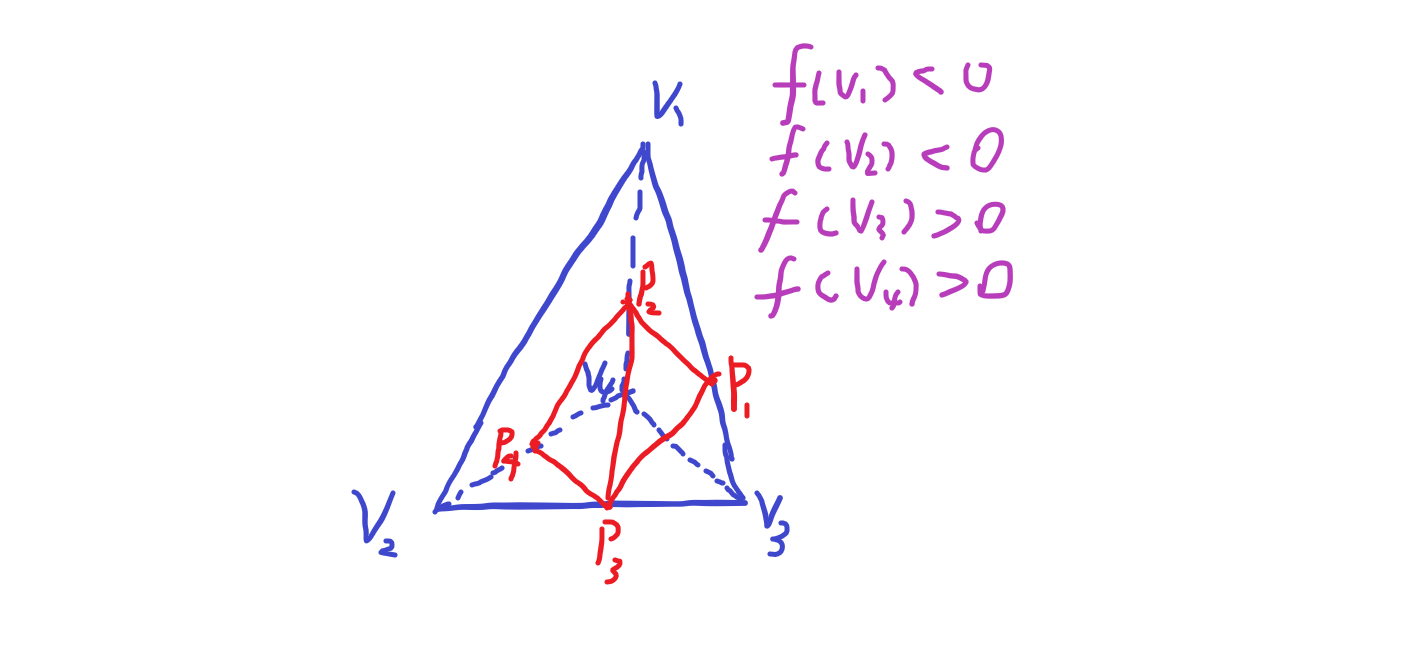上面讨论的都是单个四面体的情形，然后我们需要将一个立方体划分成六个四面体，每个四面体执行上面的Marching Tetrahedra算法，最后将每个四面体得到的三角形合起来。

这里为了验证前面水平集算法实现的正确性，将构建得到的水平集传入Marching Cube中，构建一个网格模型，即构建水平集的逆过程。重构过程根据前面水平集划分的欧拉网格大小，对每一个立方体执行Marching Cube算法，三重循环即可。

下面几张图展示了从水平集重建的网格。左边是原三角网格模型，精度均比较高，右边的是通过构建了水平集之后再从水平集重建的网格模型。受限于水平集的欧拉网格精度，重建得到的网格模型显然精度没有那么高，是一个粗糙的low poly模型。通过Marching Tetrahedra算法得到的网格依然是封闭的，这也验证了我们前面的水平集算法实现的正确性。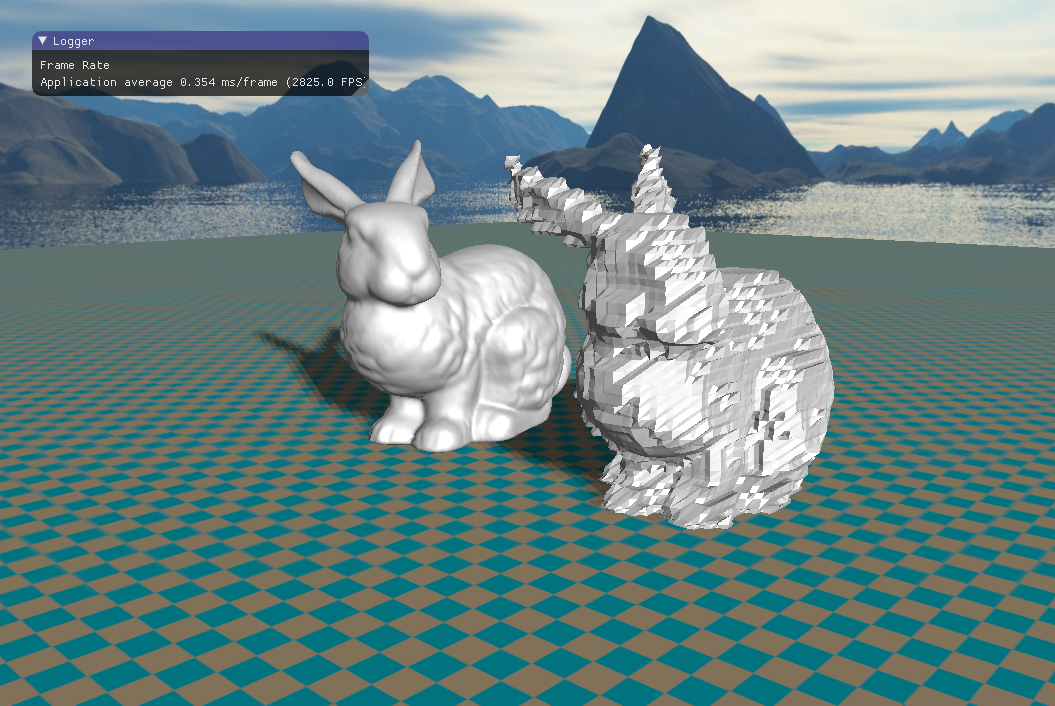## 参考资料：

$$ Edelsbrunner H, Mucke E P. Simulation of Simplicity: A Technique to Cope with Degenerate Cases in Geometric Algorithms[J]. Acm Transactions on Graphics, 1990, 9(1):66-104.

$$ 《Fluid Simulation For Computer Graphics》, Robert Bridson.